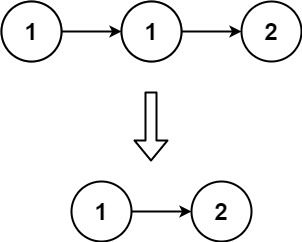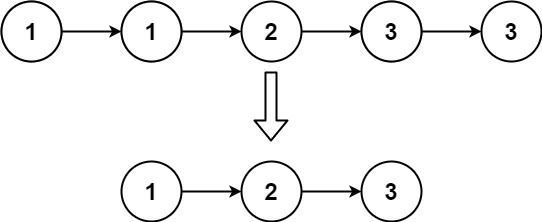## 83. Remove Duplicates from Sorted List

Given the `head` of a sorted linked list, delete all duplicates such that each element appears only once. Return the linked list sorted as well.

Example 1:```Input: head = [1,1,2]
Output: [1,2]
```

Example 2:```Input: head = [1,1,2,3,3]
Output: [1,2,3]
```

Constraints:

• The number of nodes in the list is in the range `[0, 300]`.
• `-100 <= Node.val <= 100`
• The list is guaranteed to be sorted in ascending order.

## Rust Solution

``````struct Solution;
use rustgym_util::*;

impl Solution {
while let Some(n) = p {
while let Some(m) = n.next.as_mut() {
if m.val != n.val {
break;
}
n.next = m.next.take();
}
p = n.next.as_mut();
}